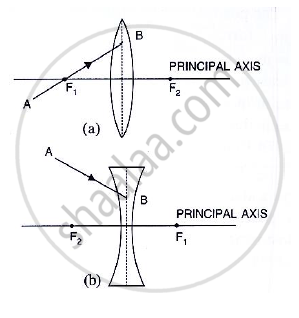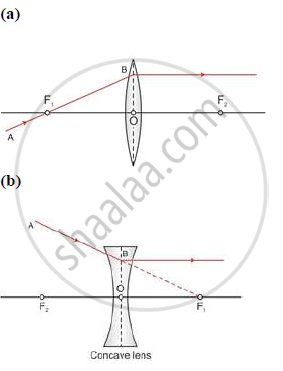Share
Notifications

View all notifications
Books Shortlist
Your shortlist is empty

# The Two Foci of the Thin Lenses Shown in Diagram (A) and (B) and Ab is the Incident Ray. Compete the Diagram to Show the Path of the Ray Ab After Refraction Through Lens in Each Diagram (A) and (B). - ICSE Class 10 - Physics

Login
Create free account

Forgot password?
ConceptConcept Refraction of Light Through a Lense

#### Question

In Fig 5.18, F1 and F2 are the two foci of the thin lenses shown in diagram (a) and (b) and AB is the incident ray. Compete the diagram to show the path of the ray AB after refraction through the lens in each diagram (a) and (b).#### SolutionIs there an error in this question or solution?

#### APPEARS IN

Solution The Two Foci of the Thin Lenses Shown in Diagram (A) and (B) and Ab is the Incident Ray. Compete the Diagram to Show the Path of the Ray Ab After Refraction Through Lens in Each Diagram (A) and (B). Concept: Concept Refraction of Light Through a Lense.
S